# Imaginary and complex numbers

Imaginary numbers. Imaginary unit. Imaginary roots.
Real numbers. Complex numbers.

x 2 = a ,

where a  – a known value. Its solution may be presented as: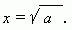Here the three cases are possible:

 1). If a = 0 , then x = 0. 2). If a is a positive number, then its square root has two values: one positive and one negative; for example, the equation x 2 = 25  has the two roots: 5 and  –5. This is often written as the root with double sign before: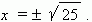3). If a – a negative number, then the equation has no solution among known us positive and negative numbers, because the second power of any number is a non-negative number (think over this!). But, if we wish to receive solutions of the equation x 2 = a also at negative values of a , we are obliged to introduce the new kind numbers – imaginary numbers. So, a number is imaginary , if its second power is a negative number. According to this definition of imaginary numbers we can define an imaginary unit as: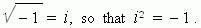Then, for the equation

x 2 =  – 25

we receive the two imaginary roots: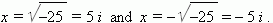Substituting both these roots into our equation we’ll receive the identity. Check it, please!

In contrast to imaginary numbers all the rest numbers (positive and negative, integers and fractional, rational and irrational ones) are called real numbers. A sum of a real and an imaginary number is called a complex number , and marked as:

a + b i  ,

where a, b –  real numbers, i –  an imaginary unit.

In more details about complex numbers see the section “Complex numbers” .

E x a m p l e s   of complex numbers:    3 + 4 i ,   7 – 13.6 i ,   0 + 25 i = 25 i ,  2 +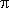i .